How to Learn in 24 Hours?The Rapid Learning Movie

 Need Help? M-F: 9am-5pm(PST): Toll-Free: (877) RAPID-10 US Direct: (714) 692-2900 Int'l: 001-714-692-2900 24/7 Online Technical Support: The Rapid Support Center Secure Online Order:Need Proof? Testimonials by Our Users

 Rapid Learning Courses: MCAT in 24 Hours (2015-16) USMLE in 24 Hours (Boards) Chemistry in 24 Hours Biology in 24 Hours Physics in 24 Hours Mathematics in 24 Hours Psychology in 24 Hours SAT in 24 Hours ACT in 24 Hours AP in 24 Hours CLEP in 24 Hours DAT in 24 Hours (Dental) OAT in 24 Hours (Optometry) PCAT in 24 Hours (Pharmacy) Nursing Entrance Exams Certification in 24 Hours eBook - Survival Kits Audiobooks (MP3)

 Tell-A-Friend: Have friends taking science and math courses too? Tell them about our rapid learning system.Home »  Mathematics »  Pre-Calculus

Right Triangle Trigonometry and Trigonometric Functions of Any Angle

 Topic Review on "Title": Right triangle: A triangle in which one angle is a right angleTrigonometric functions on a circle with radius r: For any angle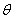in standard position, letbe the point on the terminal side ofthat is also on the circle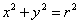.,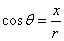,,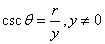,, and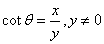.  The trigonometric functions of an acute angle: Ifis an acute angle of a right triangle, the six trigonometric functions ofare as follow: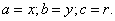,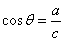,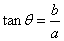,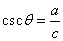,, and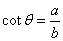.  Complementary angles: Two angles are called complementary if their sum equals to 90 degrees.  Angle of elevation: The acute angle measured from the horizontal to a line-of-sight observation of the object.  Angle of depression: The acute angle made by the line-of –sight observation of the object and the horizontal.  The trigonometric functions of any angle: Letbe an angle in standard position and let P(x,y) be a point, not the origin, on the terminal side that can be in any quadrant. If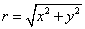is the distance from the origin to the point P(x,y), then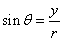,,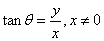,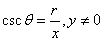,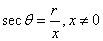, and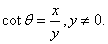Trigonometric identities: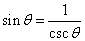,,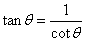,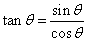and.

Rapid Study Kit for "Title":
 Flash Movie Flash Game Flash Card Core Concept Tutorial Problem Solving Drill Review Cheat Sheet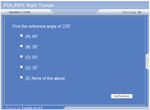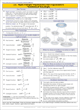"Title" Tutorial Summary : This tutorial describes right angle trigonometry in a variety of ways.  First, the trigonometric functions of acute angles are mentioned along with the complementary angle theorem. Solving right triangles is shown with the use of examples and trigonometric functions on a circle with radius r. Next, applications of trigonometric functions are shown in the examples. It is important to use problem solving techniques when dealing with trigonometry application problems. Last, the use of right triangle is used to find the trigonometric functions of any angle. Evaluating the values of a trigonometric function is shown with the use of a calculator and graphical examples.

 Tutorial Features: Specific Tutorial Features: • Graphic representation of right triangles to show the trigonometric functions. • Example problems showing the different relations between x, y and r. • Pneumonic memory device for remembering all the trigonometric functions with the respect to the sides of a right triangle. Series Features: • Concept map showing inter-connections of new concepts in this tutorial and those previously introduced. • Definition slides introduce terms as they are needed. • Visual representation of concepts • Animated examples—worked out step by step • A concise summary is given at the conclusion of the tutorial.

 "Title" Topic List: Right triangle The trigonometric functions on a circle with radius r Right angle trigonometry The trigonometric functions of an acute angle Complementary angles Co-functions Solving right triangles Applications of trigonometric functions Angle of elevation Angle of depression Trigonometric functions of any angle Trigonometric functions of a real number t Identities Evaluating the values of a trigonometric function The sign of the trigonometric functions Reference angles

See all 24 lessons in Pre-Calculus, including concept tutorials, problem drills and cheat sheets:
Teach Yourself Pre-Calculus Visually in 24 Hours